Courses

# RD Sharma Solutions -Ex-18.1(Part - 1), Surface Area And Volume Of A Cuboid And Cube, Class 9, Maths Class 9 Notes | EduRev

## Class 9 : RD Sharma Solutions -Ex-18.1(Part - 1), Surface Area And Volume Of A Cuboid And Cube, Class 9, Maths Class 9 Notes | EduRev

The document RD Sharma Solutions -Ex-18.1(Part - 1), Surface Area And Volume Of A Cuboid And Cube, Class 9, Maths Class 9 Notes | EduRev is a part of the Class 9 Course RD Sharma Solutions for Class 9 Mathematics.
All you need of Class 9 at this link: Class 9

Q1) Find the lateral surface area and total surface area of a cuboid of length 80 cm, breadth 40 cm and height 20 cm.

Solution:

Given that:

Cuboid length (l) = 80cm

Height (h) = 20cm

We know that,

Total Surface Area = 2[lb+bh+hl]

= 2[(80)(40)+(40)(20)+(20)(80)]

= 2[3200+800+1600]

= 2

= 11200 cm2

Lateral Surface Area = 2[l+b]h

= 2[80+40]20

= 40

= 4800 cm2

Q2) Find the lateral surface area and total surface area of a cube of edge 10 cm.

Solution:

Cube of edge (a) = 10 cm

We know that,

Cube Lateral Surface Area = 4 a2

= 4(10*10)

= 400 cm2

Total Surface Area = 6 a2

= 6*102

= 600 cm2

Q3) Find the ratio of the total surface area and lateral surface area of a cube.

Solution:

Total Surface Area of the Cube (TSA) = 6 a2

Where, a = edge of the cube

And, Lateral surface area of the Cube (LSA) = 4 a2

Where, a = edge of the cube

Hence, Ratio of TSA and LSA =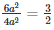is 3:2.

Q4) Mary wants to decorate her Christmas tree. She wants to place the tree on a wooden block covered with colored paper with a picture of Santa Claus on it. She must know the exact quantity of paper to buy for this purpose. If the box has length, breadth, and height as 80 cm, 40 cm and 20 cm respectively. How many square sheets of paper of side 40 cm would she require?

Solution:

Given that:

Mary wants to paste a paper on the outer surface of the wooden block. The quantity of the paper required would be equal to the surface area of the box which is of the shape of a cuboid.

The dimensions of the wooden block are:

Length (l) = 80cm

Height (h) = 20cm

Surface Area of the wooden box = 2[lb+bh+hl]

= 2[(80*40)+(40*20)+(20*80)]

= 2

= 11200 cm2

The Area of each sheet of the paper = 40×40 cm2

= 1600 cm2

Therefore, the number of sheets required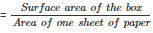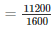= 7

So, she would require 7 sheets.

Q5) The length, breadth, and height of a room are 5 m, 4 m and 3 m respectively. Find the cost of whitewashing the walls of the room and the ceiling at the rate of Rs 7.50 m2.

Solution:

Total Area to be washed = lb+2(l+b)h

Where, length (l) = 5m

height (h) = 3m

Therefore, the total area to be white washed is = (5*4)+2*(5+4)*3

= 74 m2

Now, The cost of white washing 1 m2 is Rs. 7.50

Therefore, the cost of white washing 74 m2  = (74*7.50)

= Rs. 555/-

Q6) Three equal cubes are placed adjacently in a row. Find the ratio of a total surface area of the new cuboid to that of the sum of the surface areas of the three cubes.

Solution:

Length of the new cuboid = 3a

Breadth of the cuboid = a

Height of the new cuboid = a

The Total surface area of the new cuboid (TSA) =  2(lb+bh+hl)

• (TSA)1  =  2(3a∗a+a∗a+a∗3a)
• (TSA)1  =  14a2

The Total Surface area of three cubes

• (TSA)2  =  3∗6a2
• (TSA)2  =  18a2

Therefore,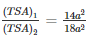Therefore,Ratiois 7:9

Q7) A 4 cm cube is cut into 1 cm cubes. Calculate the total surface area of all the small cubes.

Solution:

Edge of the cube (a) = 4cm

Volume of the cube =  a3

` =  43

=  64cm3

Edge of the cube =  1cm3

Therefore,Total number of small cubes =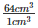= 64

Therefore,Total Surface area of all the cubes = 64∗6∗1 = 384cm2

Q8) The length of a hall is 18 m and the width 12 m. The sum of the areas of the floor and the flat roof is equal to the sum of the areas of the four walls. Find the height of the hall.

Solution:

Length of the hall = 18m

Width of the hall = 12m

Now given,

Area of the floor and the flat roof = sum of the areas of four walls

⇒2∗lb = 2∗lh+2∗bh

⇒lb = lh+bh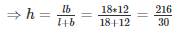= 7.2m

Q9) Hameed has built a cubical water tank with lid for his house, with each other edge 1.5 m long. He gets the outer surface of the tank excluding the base, covered with square tiles of side 25 cm. Find how much he would spend for the tiles if the cost of tiles is Rs 360 per dozen.

Solution:

Given that

Hameed is getting 5 outer faces of the tank covered with tiles, he would need to know the surface area of the tank, to decide on the quantity of tiles required.

Edge of the cubical tank (a) = 1.5m = 150cm

So, surface area of the tank =  5∗150∗150cm2

Area of each square tile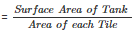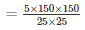= 180

Cost of 1 dozen tiles, i.e., cost of 12 tiles = Rs 360

Therefore, cost of one tile =  Rs.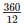= Rs.30

So, the cost of 180 tiles =  180∗30 = Rs.5400

Q10) Each edge of a cube is increased by 50%. Find the percentage increase in the surface area of the cube.

Solution:

Let ‘a’ be the edge of the cube

Therefore the surface area of the cube =  6a2

i.e., S1 = 6a2

We get a new edge after increasing the edge by 50%

The new edge =  a+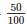∗a

=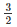∗a

Considering the new edge, the new surface area is =  6∗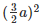i.e., S2 =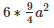S2 =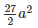Therefore, increase in the Surface Area =−6a2

=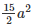So, increase in the surface area =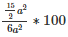=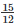∗100

= 125%

Offer running on EduRev: Apply code STAYHOME200 to get INR 200 off on our premium plan EduRev Infinity!

91 docs

,

,

,

,

,

,

,

,

,

,

,

,

,

,

,

,

,

,

,

,

,

,

,

,

,

,

,

,

,

,

;